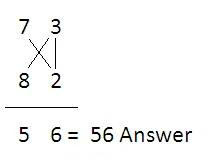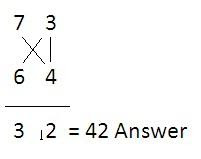# Fast Multiplication of Two Numbers - Vedic Mathematics Trick

Hi friends, in this post you will learn about fast method for multiplication of two numbers. This method is known as Vedic Mathematics method for multiplication of two numbers.

Using vertically and crosswise multiplication we can easily find the multiplication of two numbers. Using this method we can multiply two numbers which are near to 10 or 100 within few seconds. For clear understanding we will solve some examples.

Example 1: Multiply 7 × 8.

Solution: Here 7 is 3 below 10 and 8 is 2 below 10.

Therefore we write as follows:The answer is 56. Here we get 6 from 3×2 and then 5 from (8-3) or (7-2).

Important note: In case if we get two digit vertical multiplications we have to think of carry (See example).

Example 2: Multiply 7 × 6.

Solution: Here 7 is 3 below 10 and 6 is 4 below 10. So the vertical multiplication will be 12. Here we have to add ‘1’ to the next place of answer (See below).Now let us solve one more example of multiplication of numbers which are closer to 100.

Example 3: Multiply 94 × 88.

Solution: Though it looks very difficult to multiply 94 by 88, yet it is very simple because both numbers are close to 100. 94 is 6 below 100 and 88 is 12 below 100.Here we get 72 by multiplying 6 with 12 and then 82 from (88-6) or (94-12).